# SAT II Math I : Probability

## Example Questions

← Previous 1

### Example Question #1 : Probability

A penny is altered so that the odds are 3 to 2 against it coming up tails when tossed; a nickel is altered so that the odds are 4 to 3 against it coming up tails when tossed. If both coins are tossed; what are the odds of both coins coming up heads?

23 to 12 against

29 to 6 in favor

23 to 12 in favor

Even

29 to 6 against

23 to 12 against

Explanation:

3 to 2 odds in favor of heads is equal to a probability of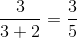, which is the probability that the penny will come up heads. Similarly, 4 to 3 odds in favor of heads is equal to a probability of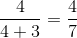, which is the probability that the nickel will come up heads.

Since the tosses of the two coins are independent, multiply the probabilities. The probability that there will be two heads is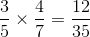This is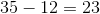to 12 against both coins coming up heads.

### Example Question #2 : Probability

A penny is altered so that the odds are 5 to 4 against it coming up tails when tossed; a nickel is altered so that the odds are 4 to 3 against it coming up tails when tossed. If both coins are tossed; what are the odds of there being one head and one tail?

32 to 31 in favor

Even

11 to 10 against

32 to 31 against

11 to 10 in favor

32 to 31 against

Explanation:

5 to 4 odds in favor of heads is equal to a probability of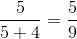, which is the probability that the penny will come up heads. The probability that the penny will come up tails is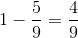.

Similarly, 4 to 3 odds in favor of heads is equal to a probability of, which is the probability that the nickel will come up heads. The probablity that the nickel will come up tails is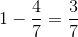.

The outcomes of the tosses of the penny and the nickel are independent, so the probabilities can be multiplied.

The probability of the penny being heads and the nickel being tails is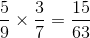; that of the reverse is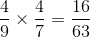.

These are disjoint, so add these probabilities; the probability that one head and one tail will come up will be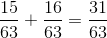. This translates to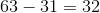to 31 odds against this event.

### Example Question #1 : Probability

A red die is altered so that it comes up a "1"of the time; the other five numbers come up with equal probability to each other. A blue die is altered similarly.

Both dice are rolled. What are the odds against rolling doubles (both numbers are the same)?

41 to 8

40 to 9

39 to 10

Insufficient information is given to solve the problem.

6 to 1

40 to 9

Explanation:

Each die comes up "1" with probability, and the probabilities of the other five rolls are equal, so the probability of a "2" on either die (or any other roll) is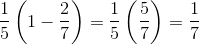.

The probability of rolling a double 1 is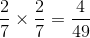.

The probability of rolling any other given double is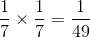.

Therefore, the probability of rolling any ofthe six doubles is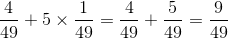,

which is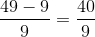odds against doubles - that is, 40 to 9.

### Example Question #1 : Probability

What is the probability of getting an ace in any standard deck of cards?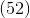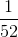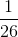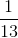Explanation:

There are four aces in a standard fifty-two card deck. So we set up a fraction.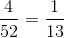The numerator represents how many possibilites we are looking for. The denominator represents the total in the given data.

Answer is.

### Example Question #5 : Probability

I have five white balls, three blue balls, and two yellow balls. What's the probability I get either white or blue?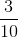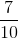Explanation:

When we see the word or, we are adding probabilities. Since we are looking for white or blue, our chance is: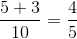The numerator represents how many possibilites we are looking for. The denominator represents the total in the given data.

Answer is.

### Example Question #6 : Probability

I have five white balls, three blue balls, and two yellow balls. What's the probability I DON'T get a blue ball?Explanation:

Since I don't want a blue ball, I just subtract the total number of balls and the number of blue balls in the set. The probability is: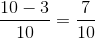The numerator represents how many possibilites we are looking for. The denominator represents the total in the given data.

Answer is.

### Example Question #7 : Probability

If there areblue balls,black balls,green balls, andred balls and I want to pick two balls without replacement. what’s the probability I get a black ball on the first pull and a red ball on the second?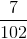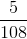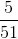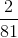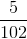Explanation:

Since we are trying to get first black then red balls without replacement, this is a condition we need to meet. This means we need to multiply probabilities. For my first pick I have five balls out of eighteen in which the probability is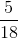. With one ball gone, there are only seventeen balls left. Since I want a red ball next, there are three left out of seventeen balls or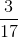. Now, we multiply our probabilities: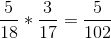### Example Question #8 : Probability

Joe has five apples, four oranges, six bananas, and five limes. What's the probability he gets two apples without replacement?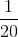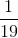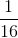Explanation:

Since we are trying to get two apples without replacement, this is a condition we need to meet. This means we need to multiply probabilities. For his first pick he has five apples out of twenty fruits in which the probability is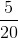or. With one apple gone, there are only nineteen fruits left. Since he wants to get another apple, there areonly four apples left out of nineteen fruits or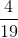. Now, we multiply our probabilities: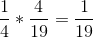### Example Question #9 : Probability

I pick a number fromto. What's the probability I get a prime number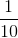Explanation:

Prime numbers are numbers with factors ofand itself. Those numbers are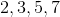. Our probability: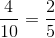The numerator represents how many possibilites we are looking for. The denominator represents the total in the given data.

Answer is.

### Example Question #10 : Probability

Sheila flips a cointimes. What’s the probability she doesn’t get heads on all the flips?To not get any heads, this would mean Sheila would need to get tails on all the flips. Since there are two choices in a flip and Sheila only want tails, our chances is. Since she needs this done twice more, this becomes a condition and we need to multiply out our probabiities. The odds are the same so our answer is just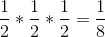.